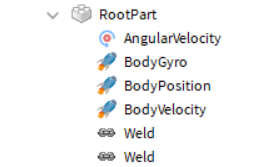# Problems with rotating a model using angular velocity

Currently I have an angular velocity parented to the root part of my model. The vector of the angular velocity is changing on key pressed, however the model doesn’t rotate at all.

Local script

``````userInputService.InputBegan:connect(function(inputObject, gameProcessedEvent)
if inputObject.KeyCode == Enum.KeyCode.W then
WHeld = true
elseif inputObject.KeyCode == Enum.KeyCode.A then
AHeld = true
elseif inputObject.KeyCode == Enum.KeyCode.S then
SHeld = true
elseif inputObject.KeyCode == Enum.KeyCode.D then
DHeld = true
end
end)

while wait(0.1) do
SubRootPart.BodyVelocity.Velocity = SubRootPart.CFrame.LookVector * SubRootPart.BodyVelocity.Velocity.Magnitude
if WHeld == true then
SubRootPart.AngularVelocity.AngularVelocity = Vector3.new(100, 0, 0)
elseif AHeld == true then

elseif SHeld == true then

elseif DHeld == true then

end
end

``````

I also have a body position within the root part with its y axis set to infinite so to prevent the model from falling, I imagine this could (maybe?) be the root cause of my problem but I’m unsure in how to stop my model from falling otherwise.1 Like

Your model needs to be unanchored and make sure your body movers can apply the neccesary force